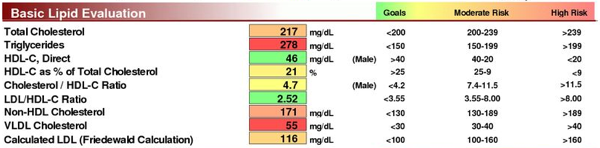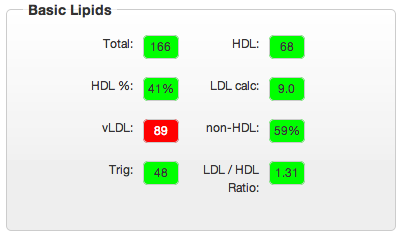# Converting A Range And A Value Into A Scaled Value, With A Nice Background Color

I’m working on a web app that receives HL7 formatted medical data through my import utility that I’ve talked about a lot, recently. Once I receive those results, I have to parse them out of the file and then display a nice little chart that shows the value of the results we care about with a color-coded indicator of health risk.

It’s essentially supposed to look like the 1st and 2nd columns of this sample data file:### The Rules And Ranges

Here’s the core set of requirements with examples:

• I have a range, which could be made of two integers or a floating point numbers, and a value within that range.
• I need to convert the range to a fixed size range (0..10) and adjust the value accordingly
• if the value is below the range, return 0.
• if the value is above the range, return 10.

For example, If I have a range of 1 through 100 with a value of 50, when I convert to range 0 through 10, the adjusted value is 5. Once I have the adjusted value, I can use a range of css classes to get the correct color for the background of the value I am displaying.

It’s not quite that simple, though. In the above picture, I’ve included the last three columns to illustrate the types of ranges that I have to deal with. Most of them are fairly straight forward ranges, but none of them are close to same. We have ranges that vary from 3.55 => 8 to 200 => 239. On top of that, some of the ranges are inverted, such as HDL-C where the range is 40 => 20, higher to lower.

### A Math Lesson: Scaling The Range And Value

To keep things simple at first, I decided to start with the normal ranges; lower number => higher number. Even at that, it took me a while to figure this out. Fortunately I had some help from David Alpert and Dean Weber in verifying what I was doing.

It’s much easier to think about the scale of one range compared to another, when we think about the size of the ranges, not the actual numbers that are contained within them. With any given range, the actual values are somewhat arbitrary. For example, a range of 0 => 50 has a size of 50. Similarly, a range of 133 => 183 also has a size of 50. If we are given a range with a size of 50, and we need to scale that down to a range with a size of 10, the scale is pretty obvious; 50/10 or 5:1.

Using the size of the range is a much easier way to look at an arbitrary range, such as the 200 => 239 range for Total Cholesterol. Instead of trying to figure out how to scale this range, we only need to figure out how to scale it’s size.

Once we have the proper scale in place, we can apply that scale to the value that we have within the original range. For example, in a range of 100 => 150, a value of 125 is halfway through the range. We also know that in our target scale of 0 => 10, 5 is the halfway point. It’s easy to infer, then, that a value of 125 in our 100 => 150 range will scale down to a value of 5 in our 0 => 10 scale. We can use this knowledge as a basic test to ensure we have the correct scaling / adjustment code.

After adjusting the range by subtracting the low end from the high end, we need to adjust the value in the same manner. That is, we need to take the value and subtract the lower end of the range. Since 150 minus 100 is 50, to give us the size of our range, we will now take our value of 125 and subtract 100, which gives us 25.

Next, we take the scale of our range compared to the target, 5:1, and reverse that into a fraction: 1/5th. If we calculate 1 / 5, we get 0.2 or 20%. We can then multiple the adjusted value of 25 by 0.2 (a.k.a 20%, a.k.a 1/5th, a.k.a 5:1 ratio). 25 * 0.2 == 5; halfway between 0 and 10, our target range. To verify, we take our adjusted value of 25 and divide it by the range size of 50. This gives us a value of 0.5 or 50%. Given our target range has a size of 10, 50% of 10 is 5.

To verify our math, let’s apply it to another range and value: range 275 => 295 and value 290. The size of this range is 295 – 275 == 20. The scale of a range of size 20 compared to the target range of 10 is 2:1, or 1/2 or 0.5. The adjusted value is 290 – 275 == 15. When we scale the adjusted value of 15 down by 0.5, we end up with 7.5, which is 75% of the way through our range size of 10. To verify, we can divide 15 by 20 (the adjusted value and range size) and we get 0.75 or 75%.

Notice that we can’t use the literal values to do the verification. If we took 125 / 150 from the first example, we would end up with 0.8333333. If we took 290 / 295 from the second example, we would end up with 0.98305. Neither of these is correct because we are not looking at a range of 0 to 150 or a range of 0 to 295. Therefore, It’s not the literal numbers that are valuable in these calculations, but the size of the range that we are dealing with and the value adjusted accordingly.

### Implementing The Math In Ruby

In spite of the long explanation of the solution, the code is fairly short. You can see the full implementation of my ruby class via the github gist that David, Dean and I used to discuss the problem. Here’s the gist of the gist, though. (In this case I chose to use the words “goal” to represent the low end of the range, and “high_risk” to represent the upper end of the range. I did this because these are the meaningful terms used in the system. Proper modeling of the domain is always a good idea).

```def score(value)
range_size = self.high_risk_above - self.goal_below

adjusted_value = range_size if value > self.high_risk_above

raw_score = (10 * value_percent)

score = raw_score.round(0).to_iend ```

Note that my code is rather verbose so that I could easily identify everything that was happening along the way. David provided a much more terse version of my solution in the comments of the gist.

We can run several tests on this to verify that is works correctly, too:

```range = RangeResults.new(:goal_below => 10, :high_risk_above => 60)

range.score(10) #=> 0
range.score(5) #=> 0
range.score(20) #=> 2
range.score(35) #=> 5
range.score(60) #=> 10
range.score(80) #=> 10```

(If anyone is really interested, I have a full set of rspec tests that provide executable proof that my code works. I can provide this via github if you want.)

### Using CSS To Determine The Cell Color

Now that I have my score calculation all set and I am reliably receiving the correct values, I need to create a way to display the correct color for the score. There were several options for this, of course. I could use back ground images to create the nice little blocks I need. I could use a dive with a background image that is a flat color and have a css border. Or I could go with pure css and set the background color, border and anything else that I need. I decided to go with the pure css approach. To do this, I created 10 classes in my css file, named “zero” through “ten”, along with a “result” class that sets up the box with border.

```p.result
+column(1)
border: 1px solid \$gray
text-align: center
p.zero
background-color: #00ff00
p.one
background-color: #33ff00
p.two
background-color: #66ff00
p.three
background-color: #99ff00
p.four
background-color: #ccff00
p.five
background-color: #ffff00
p.six
background-color: #ffcc00
p.seven
background-color: #ff9900
p.eight
background-color: #ff6600
color: #ffffff
p.nine
background-color: #ff3300
color: #ffffff
p.ten
background-color: #ff0000
color: #ffffff```

Note that I am using SASS and not straight CSS. The “+column” and “+border-radius” are SASS mixins that give me all of the CSS that I need to create a box that is 1 column wide (with a column width being defined elsewhere) and css rounded corners for my border.

### Putting It All Together Into A Nice Pretty Page

I need a way to turn 0 => 10 as integer values, into “zero” through “ten” as string values. This was easy enough:

`def i_to_s(i)  ["zero", "one", "two", "three", "four", "five", "six", "seven", "eight", "nine", "ten"][i]end `

Now that I have all of the css in place and I have the score coming back correctly to tell me what css class I need, I have to put it all together in my rails view. I’m using HAML to generate my views, which makes it fairly simple to specify a css class based on the value from a model. My model, of course, represents the lipids that I’m dealing with and each of the lipids values.

```%ul
%li
%p Total:
%p{:class => "result #{lipids.total.score}"}= lipids.total.value if lipids
%li
%p HDL:
%p{:class => "result #{lipids.hdl.score}"}= lipids.hdl.value if lipids
%li ...
```

Our actual page design does not reflect the original image that I showed above, completely. That image came from a lab results document and not from the page design for our system. Our pages do not need all of that information, only some of it, to be useful.

The end result looks like this: